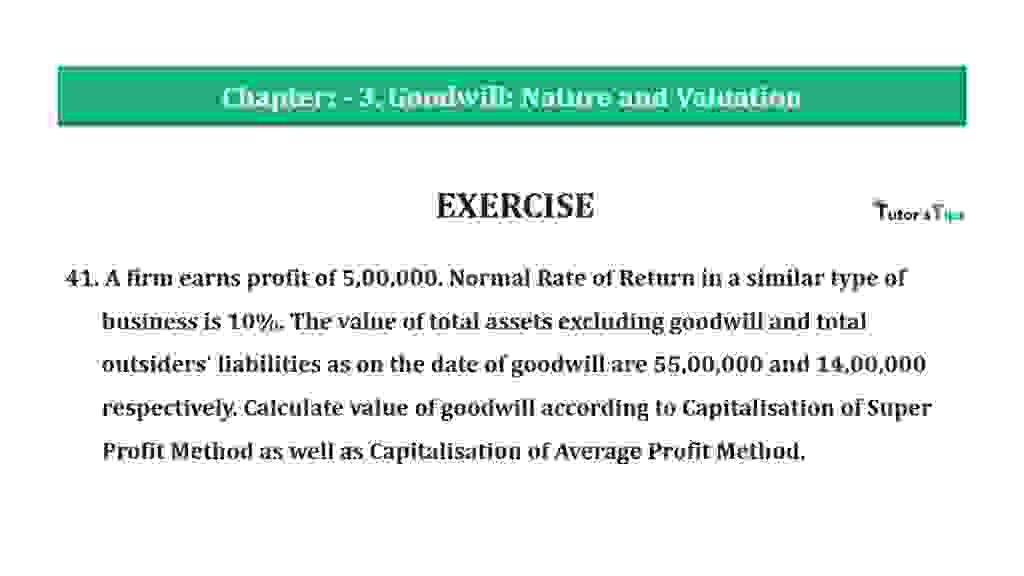# Question 41 Chapter 3 of +2-A – T.S. Grewal 12 Class Part – A Vol. 1Question 41 Chapter 3 of +2-A

41. A firm earns profit of 5,00,000. Normal Rate of Return in a similar type of  business is 10%. The value of total assets excluding goodwill and total outsiders’ liabilities as on the date of goodwill are 55,00,000 and 14,00,000 respectively. Calculate value of goodwill according to Capitalisation of Super Profit Method as well as Capitalisation of Average Profit Method.

The solution of Question 41 Chapter 3 of +2-A

:

(i) Calculation of Goodwill by Capitalisation of Super Profit Method

 Capital Employed = Total Assets – Creditors = 55,00,000 – 14,00,000 = 41,00,000
 Normal Profit = Capital Employed X Normal Rate of Return 100
 = 41,00,000 X 10 100 = 4,10,000

 Super Profit = Actual Profit – Normal Profit = 5,00,000 – 4,10,000 = 90,000

 Capitalized Value of Average Profit = Super Profit X 100 Normal Rate of Return
 = 90,000 x 100 10 = 9,00,000

(ii) Calculation of Goodwill by Capitalisation of Average Profit Method

 Capitalized Value of Profit = Average Profit X 100 Normal Rate of Return
 = 5,00,000 x 100 10 = 50,00,000

 Capital Employed = Total Assets  – Creditors = 55,00,000 – 14,00,000 = 41,00,000 Goodwill = Capitalized value of Profit – Actual Capital = 50,00,000 – 41,00,000 = 9,00,000
 Normal Profit = Capital Employed X Normal Rate of Return 100
 = 6,30,000 X 5 100 = 31,500
 Capitalized Value of Average Profit = Super Profit X 100 Normal Rate of Return
 = 90,000 x 100 10 = 9,00,000

### T.S. Grewal’s Double Entry Book Keeping +2 (Vol. I: Accounting for Not-for-Profit Organizations and Partnership Firms)

• Chapter No. 1 – Financial Statement of Not-For-Profit Organisations
• Chapter No. 2 – Accounting for Partnership Firms – Fundamentals
• Chapter No. 3 – Goodwill: Nature and Valuation
• Chapter No. 4 – Change in Profit-Sharing Ratio Among the Existing Partners
• Chapter No. 5 – Admission of a Partner
• Chapter No. 6 – Retirement/Death of a Partner
• Chapter No. 7 – Dissolution of a Partnership Firm

### T.S. Grewal’s Double Entry Book Keeping (Vol. II: Accounting for Companies)

• Chapter No. 1 – Financial Statements of a Company
• Chapter No. 2 – Financial Statement Analysis
• Chapter No. 3 – Tools of Financial Statement Analysis – Comparative Statements and Common- Size Statements
• Chapter No. 4 – Accounting Ratios
• Chapter No. 5 – Cash Flow StatementVol. I: Accounting for Not-for-Profit Organizations and Partnership Firms